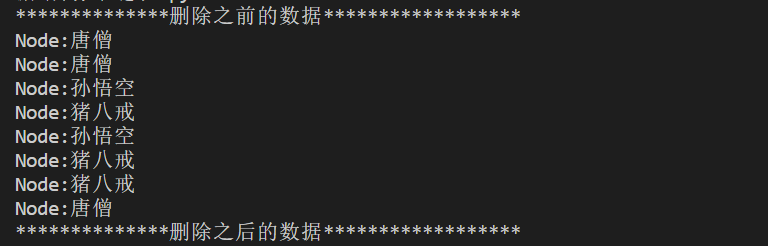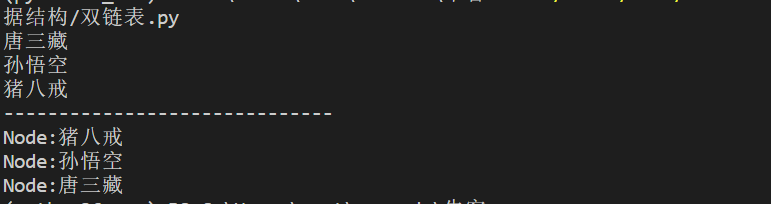⭐ 链式结构包含：node（节点）还有value（值），由于内存不连续的，那么对于数据的插入，只需找到一个节点便可以插入数据，这也是链表优于列表的一个优点，反之，对于数据的删除，由于不是连续的，不能通过索引删除数据，只能一一遍历删除元素。
⭐ 接下来上代码 单链表的增删功能

```# 定义一个结点的类
class Node():
def __init__(self,value=None,next=None):
self.next = next
self.value = value

def __str__(self):
return "Node:{}".format(self.value)

# 定义一个连接点的类

def __init__(self):
self.root = Node()
self.size = 0
self.next = None  # 增加新数据时，将信数据的地址与谁关联

# 只要是追加 就要创造节点
def append(self,value):
node = Node(value)
# 判断root节点下面是否有数据
if not self.next: # 如果没有节点
self.root.next = node  # 将新节点挂在root后面
else:
self.next.next = node  # 将新节点挂在最后一个节点上
self.next = node # 重新赋值 一开始的是None
self.size += 1

def append_first(self,value):
# 只要是追加 就要创造节点
node = Node(value)
# 判断root下面是否存在节点
if not self.next:
self.root.next = node
self.next = node
else:
temp = self.root.next # 获取原来root后面的那个节点
self.root.next = node #  将新节点挂在root后面
node.next = temp # 新的节点的下一个节点是原来的root的节点

# 迭代链表
def __iter__(self):
# 获得root节点下面的数据
current = self.root.next
# 判断current是否有之 由于一开始赋予value和next没有值 所以进行判断 否则报错
if current:
# 判断时候有下一个节点 有的话则返回下一个结点的值
while current is not self.next: # self.next可以列结成最后一个节点
yield current
current = current.next
yield current

# 查找数据
def find(self,value):
for n in self.__iter__():
if n.value == value:
return n

# 查找出现的次数
def find_count(self,value):
count = 0
for n in self.__iter__():
if n.value == value:
count += 1
return count

# 移除数据(链表的移除，需要逐一遍历，找到当前元素的节点，再删除)
def remove(self,value):
prve = self.root
for n in self.__iter__():
# 判断节点的值与要删除的值是否相等
if n.value == value:
# 查看是不是最后一个节点
if n == self.next:
# 更新倒数第二节点的关系
prve.next = None
# 更新最后一个节点为原倒数第二个节点
self.next = prve
prve.next = None
# 删除当前节点
del n
# 链表大小减少
self.size -= 1
return True
prve = n

def remove_all(self,value):
prve =self.root
for n in self.__iter__():
if n.value == value:
if n == self.next:
prve.next = None
self.next = prve
prve.next = n.next
# 删除当前节点
del n
# 链表大小减少
self.size -= 1
return True
prve = n

if __name__ == "__main__":
#     print(v)

print("**************删除之前的数据******************")
print(v)

print("**************删除之后的数据******************")
print(v)        ```⭐ 接下来上代码 双链表的增删功能：

```# 定义一个结点的类
class Node():
def __init__(self,value=None,node=None,next=None):
self.value = value
self.node = node
self.next = next
def __str__(self):
return "Node:{}".format(self.value)

# 定义一个连接点的类
def __init__(self):
self.root = Node()
self.size = 0
self.end = None

def append(self,value):
node = Node(value)
# 判断root节点下面是否有数据
if not self.end: # 如果没有元素
self.root.next = node  # 将root 的下一个节点 设置为新的node节点
node.prev = self.root  # 设置新节点的 上一个节点 为 root
else:
self.end.next = node  # 将原来最后节点的下一个节点 设置为新的node节点
node.prev = self.end # 设置新节点的 上一个节点 为 原来的最后一个节点
self.end = node # 更新最后 一个节点新加的node节点
self.size += 1

def append_first(self,value):
node = Node(value) # 封装节点对象
# 判断是否已经有数据
if not self.end:# 如果没有元素
self.end = node # 更新最后 一个节点新加的node节点
else:
# temp指向node，node指向root，root指向temp
temp = self.root.next # 保存原来的第一个节点
node.next = temp # 设置新节的下一个节为原来的 第一个节点
temp.prev = node # 更新原来的第一个节点的上一个节点位置为 新的node节点
node.prev = self.root # 设置新节点的 上一个节点 为 root
self.root.next = node # 将root 的下一个节点 设置为新的node节点
self.size += 1

# 正序循环
def __iter__(self):
current = self.root.next
if current:
while current is not self.end:
yield current.value
current = current.next
yield current.value

# 倒叙循环
def revers_iter(self):
current  = self.end #获取最后一节点
if current:
while current is not self.root:
yield current
current = current.prev

if __name__ == "__main__":